# Rectangle Overlap

 Important You are given two rectangles, each defined by an upper left (UL) corner and a lower right (LR) corner. Both rectangles’ edges will always be parallel to the x or y axis, as shown in Figure 11-2. Write a function that determines whether the two rectangles overlap. The function should return 1 if the rectangles overlap and 0 if they do not. For convenience, you may use the following classes: ` class Point { public int x; public int y; public Point( int x, int y ){ this.x = x; this.y = y; } } class Rect { public Point ul; public Point lr; public Rect( Point ul, Point lr ){ this.ul = ul; this.lr = lr; } }`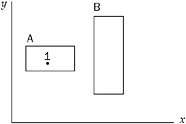Figure 11-2 The method should take two Rect objects and return true if they overlap, false if they don’t.

Before you jump into the problem, it’s important to work out a few properties about rectangles and their vertices. First, given the upper left (UL) point and lower right (LR) corners, it is not difficult to get the upper right (UR) and lower left (LL) corners. The coordinates of the upper right corner are the upper left’s y and the lower right’s x. The lower left corner is at the upper left’s x and the lower right’s y.

It is also useful to be able to determine whether a point falls inside a rectangle. A point is inside a rectangle if the point’s x is greater than the rectangle’s UL corner’s x and less than the rectangle’s LR corner’s x, and the point’s y is greater than the rectangle’s LR corners’s y and less than the rectangle’s UL corner’s y. You can see this in Figure 11-2, where point 1 is inside rectangle A. Now we can move on to the problem.

This problem seems pretty straightforward. Start by considering the ways in which two rectangles can overlap. Try to divide the different ways into various cases. A good place to begin is by examining where the corners of a rectangle end up when it overlaps another. Perhaps you could enumerate the ways in which two rectangles can overlap by counting the number of corners of one rectangle that are inside the other rectangle. The cases that you must consider are when one of the rectangles will have 0, 1, 2, 3, or 4 corners inside the other. Take these cases one at a time. Begin by considering a case in which no corners of either rectangle are inside the other. This is illustrated in Figure 11-3.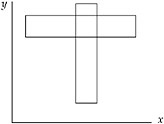Figure 11-3

Consider what conditions have to be true for two rectangles to overlap without having any corners inside each other. First, the wider rectangle must be shorter than the narrower rectangle. Next, the two rectangles must be positioned so the overlap occurs. This means that the narrower rectangle’s x coordinates must be between the wider rectangle’s x coordinates and the shorter rectangle’s y coordinates must be between the taller rectangle’s y coordinates. If all of these conditions are true, you have two rectangles that overlap without having any corners inside of each other.

Now consider the second case, in which rectangles may overlap with one corner inside the other. This is illustrated in Figure 11-4. This case is relatively easy. You can simply check whether any of the four corners of one rectangle are inside the other rectangle.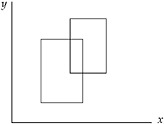Figure 11-4

In the third case, the rectangles may overlap if two points of one rectangle are inside the other. This occurs when one rectangle is half in and half out of the other rectangle, as illustrated in Figure 11-5. Here, one rectangle has no corners inside the other, and one rectangle has two corners inside the other. If you check the corners of the rectangle with no corners inside the other, you will not find overlap. If you check the rectangle with two corners overlapping, you must check at least three corners to determine overlap. However, you can’t determine ahead of time which rectangle will have no corners inside the other. Therefore, you must check three corners of each rectangle to test for overlap properly.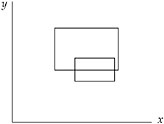Figure 11-5

The three-point case is very simple: It’s just not possible. No matter how you draw the rectangles, you can’t arrange them so that one rectangle has exactly three corners inside the other.

The four-corner case is possible. This happens if one rectangle completely subsumes the other, as shown in Figure 11-6. If you check one corner of both rectangles, you can correctly determine overlap in this case.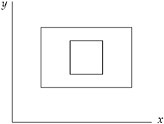Figure 11-6

Now, put your tests for determining overlap in the zero-corner, one-corner, two-corner, and four-corner cases together to encompass all of these cases. These tests check the widths, heights, and positions of both rectangles, the four corners of one rectangle, the three corners of each rectangle, and the one corner of each rectangle, respectively. You could test each of these cases individually, but that’s very repetitive. Instead, try to develop a single test that encompasses all of these cases. Start by checking the widths, heights, and positions of both rectangles to cover the zero-corner case. Next, check the four corners of one rectangle to cover the one-corner case. Then, to include the two-corner case, you’ll also have to check three corners of the other rectangle. Luckily, the four-corner case is already covered if you check four corners of one rectangle and three of the other because you’re clearly checking one corner of each. The composite test to determine rectangle overlap is to check the following:

• The heights, widths and positions of both rectangles

• Whether any of four corners of one rectangle are inside the other

• Whether any of three corners from the second rectangle are inside the first

This solution to test for overlap is correct, but it seems inefficient. It checks the heights, widths, and positions of both rectangles as well as seven of eight possible corners - and each corner check requires four comparisons. This results in 34 comparisons to calculate the answer.

Perhaps there is a better solution. Another way to think about the problem is to consider when the rectangles don’t overlap, as opposed to when they do overlap. If you know when the rectangles don’t overlap, you know when they do overlap. The conditions for not overlapping are much more straightforward. Call the two rectangles A and B. A and B do not overlap when A is above B, or A is below B, or A is to the left of B, or A is to the right of B. It is possible for more than one of these conditions to be true at the same time. For example, A could be above and to the right of B. If any one of these conditions is true, the two rectangles do not overlap. The specifics of these conditions can be summarized as follows.

The two rectangles do not overlap when

• A’s UL’s x value is greater than B’s LR’s x value or

• A’s UL’s y value is less than B’s LR’s y value or

• A’s LR’s x value is less than B’s UL’s x value or

• A’s LR’s y value is greater than B’s UL’s y value.

This solution is much simpler, requiring only four comparisons and one negation. You can implement the function as follows:

` boolean overlap( Rect a, Rect b ){     return !( a.ul.x > b.lr.x ||               a.ul.y < b.lr.y ||               a.lr.x < b.ul.x ||               a.lr.y > b.ul.y ); }`

This function works, but you can do even better. It’s possible to get rid of the logical NOT. A bit of logic theory called DeMorgan’s Law may be helpful here. This law states the following: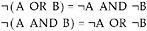Tip The symbol ¬ means NOT in the logic world.

In addition, you should recognize that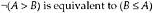Working through these rules, you’ll get the following function:

` boolean overlap( Rect a, Rect b){     return( a.ul.x <= b.lr.x &&             a.ul.y >= b.lr.y &&             a.lr.x >= b.ul.x &&             a.lr.y <= b.ul.y ); }`

To ensure that you didn’t make a mistake, it’s a good idea to verify that these conditions make sense. The preceding function determines that two rectangles overlap if

• A’s left edge is to the left of B’s right edge and

• A’s upper edge is above B’s bottom edge and

• A’s right edge is to the right of B’s left edge and

• A’s bottom edge is below B’s upper edge.

These conditions mean that rectangle B cannot be outside of rectangle A, so there must be some overlap. This makes sense.

### Big-endian or Little-endian

 Important Write a function that determines whether a computer is big-endian or little-endian.

This problem tests your knowledge of computer architectures as much as it tests your ability to program. The interviewer wants to know whether you are familiar with the term endian. If you are familiar with it, you should define it or at least try to point out the differences between big-endian and little-endian, even if you forget which is which. If you are not familiar with the term, you’ll have to ask the interviewer to explain it.

Endianness refers to the order in which a computer stores the bytes of a multibyte value. (Or, technically, the units of a multiunit value - for example, the computer may use a 16-bit unit size instead of an 8-bit unit size. We restrict ourselves to 8-bit units for simplicity, however.) Almost all modern computers use multibyte sequences to represent certain primitive data types.

For example, an integer is usually 4 bytes. The bytes within an integer can be arranged in any order, but they are almost always either least-significant byte (LSB) to most-significant byte (MSB) or MSB to LSB. Significance refers to the place value a byte represents within a multibyte value. If a byte represents the lowest place values in a (two-byte) word the byte is the LSB. For example, in the number 5A6C, 6C is the LSB. Conversely, if a byte represents the highest place values in the word, it is the MSB. In the 5A6C example, 5A is the MSB.

In a big-endian machine the MSB has the lowest address; in a little-endian machine the LSB has the lowest address. For example, a big-endian machine stores the 2-byte hexadecimal value A45C by placing A4 in the first byte and 5C in the second. In contrast, a little-endian machine stores 5C in the first byte and A4 in the second.

Endianness is important to know when reading or writing data structures, especially across networks, so that different applications can communicate with each other. Sometimes the endianness is hidden from the developer: Java uses a fixed endianness to store data, regardless of the underlying platform’s endianness, so data exchanges between two Java applications won’t normally be affected by endianness. But other languages, C in particular, don’t specify an endianness for data storage, leaving the implementation free to choose the endianness that works best for the platform. C is used to solve this problem.

To answer the problem, you have to choose some multibyte data type to work with. It’s not important which one you choose, just that the type is more than one byte. A 32-bit integer is a good choice. You need to determine how you can test this integer to figure out which byte is LSB and which is MSB. If you set the value of the integer to 1, you can distinguish between the MSB and the LSB because in an integer with the value 1, the LSB has the value 1 and the MSB has the value 0.

Unfortunately, it’s not immediately clear how to access the bytes of an integer. You might try using the bit operators because they allow access to individual bits in a variable. However, they are not particularly useful because the bit operators act as if the bits are arranged in order from least-significant bit to most-significant bit. For example, if you use the shift left operator to shift the integer 8 bits, the operator works on the integer as if it were 32 consecutive bits regardless of the true internal byte order. This property prevents you from using the bit operators to determine byte order.

How might you be able to examine the individual bytes of an integer? A character is a single-byte data type. It could be useful to view an integer as four consecutive characters. To do this, you create a pointer to the integer. Then, you can cast the integer pointer to a character pointer. This enables you to access the integer like an array of 1-byte data types. Using the character pointer, you can examine the bytes and determine the format.

Specifically, to determine the computer’s endianness, get a pointer to an integer with the value of 1. Then, cast the pointer to a char *. This changes the size of the data to which the pointer points. When you dereference this pointer you access a 1-byte character instead of a 4-byte integer. Thus, you can test the first byte and see if it is 1. If the byte’s value is 1, then the machine is little-endian because the LSB is at the lowest memory address. If the byte’s value is 0, then the machine is big-endian because the MSB is at the lowest memory address. In outline form, here is the procedure:

` Set an integer to 1 Cast a pointer to the integer as a char * If the dereferenced pointer is 1, the machine is little-endian If the dereferenced pointer is 0, the machine is big-endian`

The code for this test is as follows:

` /* Returns true if the machine is little-endian, false if the  * machine is big-endian  */ bool endianness(){     int   testNum;     char *ptr;     testNum = 1;     ptr = (char *) &testNum;     return (*ptr); /* Returns the byte at the lowest address */ }`

This solution is sufficient for an interview. However, as the goal of an interview is not just to solve problems, but also to impress your interviewer, you may want to consider a slightly more elegant way to solve this problem. It involves using a feature of C/C++ called union types. A union is like a struct, except that all of the members are allocated starting at the same location in memory. This enables you to access the same data with different variable types. The syntax is almost identical to a struct. Using a union, the code is as follows:

` /* Returns true if the machine is little-endian, false if the  * machine is big-endian  */ bool endianness(){     union {         int theInteger;         char singleByte;     } endianTest;     endianTest.theInteger = 1;     return endianTest.singleByte; }`

### Number of Ones

 Important Write a function that determines the number of 1 bits in the computer’s internal representation of a given integer.

This problem may at first sound like a base conversion problem in which you have to design an algorithm to convert a base 10 number to a two’s complement binary number. That approach is circuitous because the computer already internally stores its numbers in two’s complement binary. Instead of doing a base conversion, try counting the 1’s directly.

You can count the number of 1’s by checking the value of each bit. Ideally, you’d like to use an operator that would tell you the value of a specified bit. That way, you could iterate over all of the bits and count how many of them were 1’s. Unfortunately, this ideal operator doesn’t exist.

You can begin by trying to create a procedure that determines the value of each bit using the existing bit operators. Focus on figuring out a way to get the value of the lowest bit. One way to do this is to AND the given integer with the value 1. Let’s use 8-bit integers to keep our examples manageable, in which case 1 is stored as 00000001. The result would be either 00000000 if the given integer’s lowest bit had the value 0, or 00000001 if the given integer’s lowest bit had the value 1. In general, you can get the value of any bit if you create the correct mask. In this case, the mask is an integer with all the bits set to 0 except the bit you’re checking, which is set to 1. When you AND a mask with the value you’re checking, the result is either a 0, indicating that the bit you are checking has the value 0, or a non-zero result, indicating that the bit you are checking has the value 1.

You could create a mask for each of the bits and count the number of 1 bits. For example, the first mask would be 00000001, followed by masks of 00000010, 00000100, 00001000, and so on. This would work, but your interviewer probably doesn’t want to watch you write out that many masks. Consider the differences between each mask. Each mask is the same as the previous mask, but the 1 bit is moved one place to the left. Instead of predefining your masks, you can construct them using the shift left operator. Simply start with a mask of 00000001 and repeatedly shift the integer one bit to the left to generate all the necessary masks. This is a good technique, and if you work it out to its conclusion, it yields an acceptable answer. However, there’s a prettier and slightly faster solution that uses only one mask.

Think about what you can do with a single mask. You are trying to examine each bit of the integer, so you need to mask a different bit on each iteration. So far, you’ve been accomplishing this by shifting the mask and keeping the integer in place, but if you shifted the integer, you could examine all of its bits using the same mask. The most natural mask to use is 00000001, which yields the least-significant bit. If you keep shifting the integer right, each bit will eventually become the rightmost bit. Try working through 00000101 as an example. The rightmost bit is 1 so you would add 1 to your count and shift the integer right, yielding 00000010. This time the rightmost bit is 0. Shifting right again produces 00000001. The least significant bit in this integer is 1, so you would increment your count to 2. When you shift right a third time, the integer becomes 00000000. When the integer’s value reaches zero there are no 1 bits remaining, so you can stop counting. As in this example, you may not have to iterate through all the bits to count all the 1’s, so in many cases this algorithm is more efficient than the multiple mask algorithm. In outline, the single mask algorithm is as follows:

` Start with count = 0 While the integer is not 0     If the integer AND 1 equals 1, increment count     Shift the integer one bit to the right Return count`

Finally, check for any error cases in this code; you’ll want to look for problems with positive numbers, negative numbers, and zero. If the integer has the value of 0, the algorithm immediately and correctly returns that there are zero 1’s in the binary representation. Now consider the case where you are passed a negative number. The number will be shifted to the right, but the new bit added on the left will depend on how the shift operator treats negative values. Let’s avoid the problem entirely and use Java’s >>> operator for our example. In the other C-like languages, you can also avoid the problem by reading the value as an unsigned integer. (That solution doesn’t work with Java because there are no unsigned integer types in Java.) Using either the >>> or an unsigned integer means that the shift operator will not sign extend, and the new bits that are added during the right shifting will be 0’s. The number will eventually become all 0’s. Finally, consider the case where you are given a positive integer. This is the sample case that you worked with, and the algorithm works correctly here.

The code for this algorithm is as follows:

` int numOnesInBinary( int number ) {      int numOnes = 0;      while( number != 0 ){           if( ( number & 1 ) == 1 )               numOnes++;           number = number >>> 1;      }       return numOnes; }`

What’s the running time of this function? The function will iterate through the while loop until all the 1’s have been counted. In the best case, the given integer is 0, and the function never executes the while loop. In the worst case, this is O(n), where n is the size, in bits, of an integer.

Unless you’re incredibly good at bitwise operations, this is the best solution you’re likely to come up with in an interview. There are better solutions, though. Consider what happens at the bit level when you subtract 1 from a number. Subtracting 1 produces a value that has all the same bits as the original integer except that all the low bits up to and including the lowest 1 are flipped. For example, subtracting 1 from the value 01110000 results in the value 01101111.

If you apply the AND operation to the integer and the result of the subtraction, the result is a new number that is the same as the original integer except that the rightmost 1 is now a 0. For example, 01110000 AND (01110000 – 1) = 01110000 AND 01101111 = 01100000.

You can count the number of times that you can perform this process before the integer’s value reaches 0. This is the number of 1’s in the computer’s representation of the number. In outline form this algorithm is as follows:

` Start with count = 0 While the integer is not zero     AND the integer with the integer – 1     Increment count Return count`

Here is the code:

` int numOnesInBinary( int number ){      int numOnes = 0;      while( number != 0 ){           number = number & (number – 1);           numOnes++;      }      return numOnes; }`

This solution has a running time of O(m), where m is the number of 1’s in the solution. There may be even better solutions. Keep in mind that this solution was presented for interest, and the first solution is all that would be expected in an interview.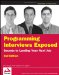Programming Interviews Exposed: Secrets to Landing Your Next Job, 2nd Edition (Programmer to Programmer)
ISBN: 047012167X
EAN: 2147483647
Year: 2007
Pages: 94

Similar book on Amazon

flylib.com © 2008-2017.
If you may any questions please contact us: flylib@qtcs.net